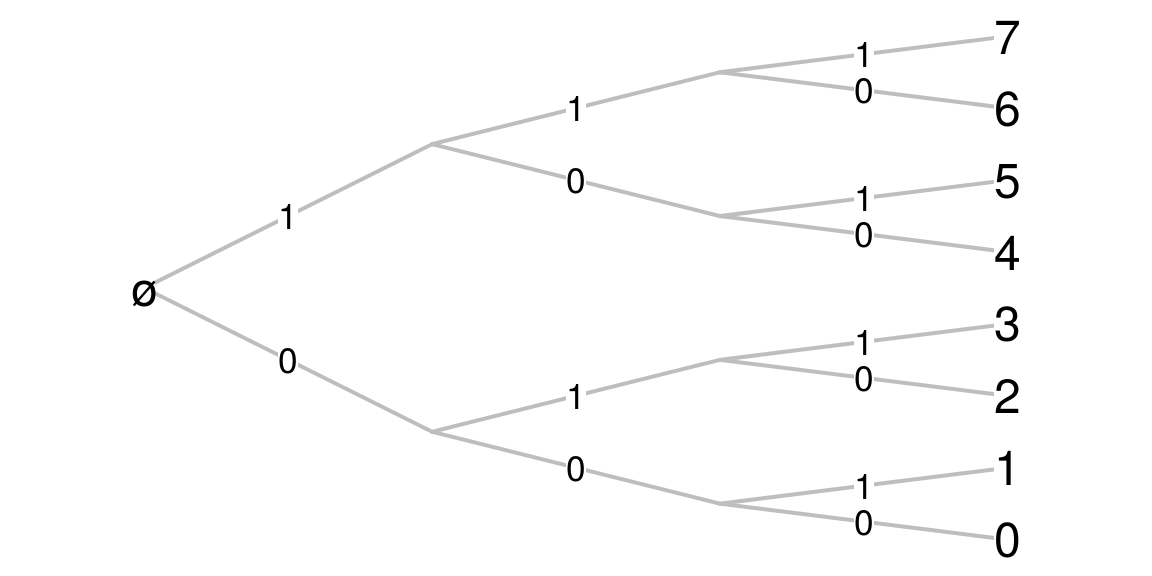1. Avoiding nested loops with a Cantor expansion

10/08/2015
Source

(first version)

I added an answer to a question on Stackoverflow about the problem of a variable amount of nested loops. But an answer to a four years old question on Stackoverflow rarely receives some attention.

Cantor expansions of integers

My answer is based on a Cantor expansion of the integers. The binary representation of integer numbers is well-known. In R, use this function to get it:

number2binary <- function(number, noBits=1+floor(log2(max(number,1)))) {
if(noBits < 1+floor(log2(number))) warning(sprintf("noBits=%s is not enough", noBits))
return( as.numeric(intToBits(number))[1:noBits] )
}
number2binary(5)
##  1 0 1
number2binary(5, noBits=4)
##  1 0 1 0

The second argument, noBits, allows to fix the length of the binary representation, adding some 0 to the right to reach it.

The binary representation is a system of numerotation. The correspondence between the usual decimal representation and the binary representation is the following:

nmax <- 10
data.frame(
N=0:nmax,
expansion=vapply(0:nmax, function(i) paste(myutils::number2binary(i), collapse=""), character(1))
)
##     N expansion
## 1   0         0
## 2   1         1
## 3   2        10
## 4   3        11
## 5   4       100
## 6   5       101
## 7   6       110
## 8   7       111
## 9   8      1000
## 10  9      1001
## 11 10      1010

The numerotation for the $2^n$ first integers is also given by the Cartesian product of the set $\{0,1\}$ with itself $n$ times:

expand.grid(epsilon0=c(0,1), epsilon1=c(0,1), epsilon2=c(0,1))
##   epsilon0 epsilon1 epsilon2
## 1        0        0        0
## 2        1        0        0
## 3        0        1        0
## 4        1        1        0
## 5        0        0        1
## 6        1        0        1
## 7        0        1        1
## 8        1        1        1

Thus, one can view the binary representation of a number $n$ as the $n$-th element of this Cartesian product (for the colexicographic order). The enumeration is easily viewed on a tree:Well, everybody knows that. In mathematical terms, the binary representation allows to write any natural number $N$ as a finite sequence of digits $\epsilon_i \in \{0,1\}$: $N = \text{"}\epsilon_0\epsilon_1\epsilon_2\ldots\epsilon_n\text{"}.$ and that means $N = \epsilon_0 + \epsilon_1\times 2 + \epsilon_2 \times 2^2 + \ldots + \epsilon_n \times 2^n.$

Such a representation is more generally possible with digits $\epsilon_i \in \{0,\ldots, r_n-1\}$, where $(r_n)$ is a sequence of integer numbers $\geq 2$, and then the representation means $N = \epsilon_0 + \epsilon_1\times \ell_1 + \epsilon_2 \times \ell_2 + \ldots + \epsilon_n \times \ell_n,$ where $\ell_n=\prod_{i=0}^{n-1} r_i$, and then this expansion of $N$ is called its $(r_n)$-ary expansion. The binary expansion is the case when $r_n\equiv 2$. The general $(r_n)$-ary expansion is called a Cantor’s number system. There also are, beyond the scope of this post, more general systems of numeration.

For example, the $(3,4,5)$-ary representation of a number $n$ is the $n$-th element of this Cartesian product $\{0,1,2\}\times\{0,1,2,3\}\times\{0,1,2,3,4\}$.

One gets the integer $N$ from its $(r_n)$-ary expansion by simply applying the above formula. Conversely, the derivation of the $(r_n)$-ary from an integer is given by the greedy algorithm:

• While $N>0$
• Take the first index $k$ such that $\ell_k > N$
• Set $\epsilon_k$ to be the Euclidean quotient of $N$ by $\ell_{k-1}$, and update $N$ to be the remainder

This R function returns the $(r_n)$-ary expansion, using the binary expansion as the default one:

intToAry <- function(n, sizes=rep(2, 1+floor(log2(max(n,1))))){
l <- c(1, cumprod(sizes))
epsilon <- numeric(length(sizes))
while(n>0){
k <- which.min(l<=n)
e <- floor(n/l[k-1])
epsilon[k-1] <- e
n <- n-e*l[k-1]
}
return(epsilon)
}
aryToInt <- function(epsilon, sizes=rep(2, length(epsilon)-1)){
sum(epsilon*c(1,cumprod(sizes[1:(length(epsilon)-1)])))
}

As an example:

intToAry(29, c(3, 4, 5))
##  2 1 2

means that $29 = 2\times 1 + 1 \times 3 + 2 \times (3\times 4)$.

Note that the last element of sizes, here 5, is not visible in the expansion. It only fixes the maximal value of $\epsilon_2$: the $(3,4,5)$-ary expansion of $N$ is $N = \epsilon_0 + \epsilon_1 \times 3 + \epsilon_2 \times (3\times 4)$ with $\epsilon_0 \in \{0,1,2\}$, $\epsilon_1 \in \{0, 1, 2, 3\}$, $\epsilon_2 \in \{0, 1, 2, 3, 4\}$.

Application to nested loops

Assume you have a nested loop:

for(i in 1:3){
for(j in 1:4){
for(k in 1:5){
doSomething(c(i,j,k))
}
}
}

This nested loop can be reduced to only one loop with the $(3,4,5)$-ary representation:

for(n in 1:(3*4*5)){
doSomething(intToAry(n)+1, c(3,4,5))
}

A Rcpp implementation

Using the intToAry function in a long loop could be time-consuming. We give below a C++ implementation for R, using the Rcpp and inline packages.

library(inline)
src <- 'int n = as<int>(N);
std::vector<int> s = as< std::vector<int> >(sizes);
std::vector<int> epsilon (s.size());
std::vector<int>::iterator it;
it = s.begin();
it = s.insert ( it , 1 );
int G[s.size()];
std::partial_sum (s.begin(), s.end(), G, std::multiplies<int>());
int k;
while(n>0){
k=1;
while(G[k]<=n){
k=k+1;
}
epsilon[k-1] = (int)n / G[k-1];
n = n % G[k-1];
}
return wrap(epsilon); '
intToAry_Rcpp <- cxxfunction(signature(N="integer",
sizes="integer"),
body=src, plugin="Rcpp")
intToAry_Rcpp(29, c(3,4,5))
##  2 1 2

Benchmarks

The C++ implementation is clearly faster.

L <- vector(mode="list", length=2*3*4*5*6*7*8*9)
system.time(
for(n in 1:(2*3*4*5*6*7*8*9)){
L[[n]] <- intToAry(n-1, c(2,3,4,5,6,7,8,9))
}
)
##    user  system elapsed
##  14.429   0.000  14.431
system.time(
for(n in 1:(2*3*4*5*6*7*8*9)){
L[[n]] <- intToAry_Rcpp(n-1, c(2,3,4,5,6,7,8,9))
}
)
##    user  system elapsed
##   1.521   0.000   1.530

A Javascript implementation

function intToAry(n, sizes) {
if (n<0) throw new Error("n must be a nonnegative integer");
for (i = 0; i<sizes.length; i++) if (sizes[i]!=parseInt(sizes[i]) || sizes[i]<1){  throw new Error("sizes must be a vector of integers be >1"); };
for (var G = , i = 0; i<sizes.length; i++) G[i+1] = G[i] * sizes[i];
if (n>=_.last(G)) throw new Error("n must be <" + _.last(G));
for (var epsilon=[], i=0; i < sizes.length; i++) epsilon[i]=0;
while(n > 0){
var k = _.findIndex(G, function(x){ return n < x; }) - 1;
var e = (n/G[k])>>0;
epsilon[k] = e;
n = n-e*G[k];
}
return epsilon;
}
intToAry(29, [3, 4, 5])
## 2,1,2